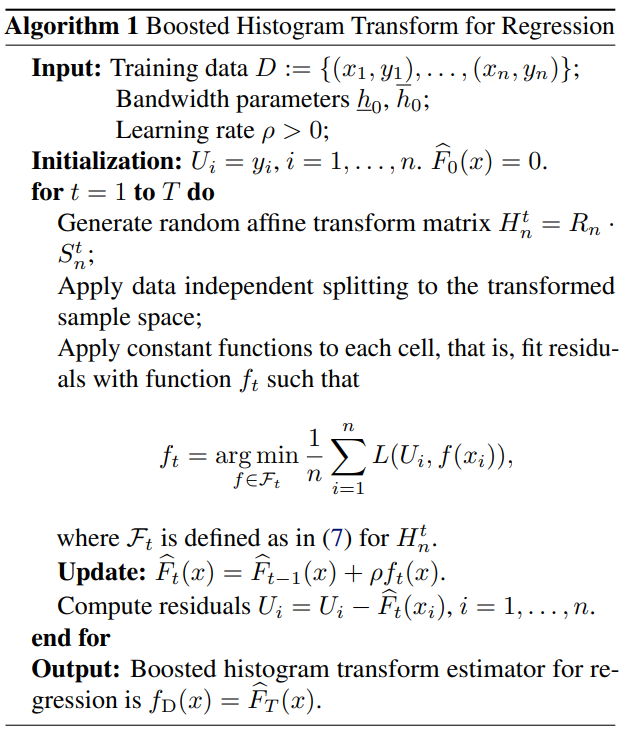# Boosted Histogram Transform for Regression### Abstract

In this paper, we propose a boosting algorithm for regression problems called boosted histogram transform for regression (BHTR) based on histogram transforms composed of random rotations, stretchings, and translations. From the theoretical perspective, we first prove fast convergence rates for BHTR under the assumption that the target function lies in the spaces C 0,α. Moreover, if the target function resides in the subspace C 1,α, for the first time we manage to explain the benefits of the boosting procedure, by establishing the upper bound of the convergence rate for the boosted regressor, i.e. BHTR, and the lower bound for base regressors, i.e. histogram transform regressors (HTR). In the experiments, compared with other state-of-the-art algorithms such as gradient boosted regression tree (GBRT), Breiman’s forest, and kernel-based methods, our BHTR algorithm shows promising performance on both synthetic and real datasets.

Type
Publication
International Conference on Machine Learning